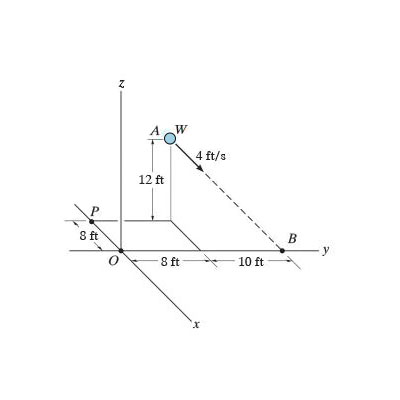# Determine the x, y, and z components of the angular momentum H_O of the particle about point O...

## Question:

Determine the {eq}x, y {/eq} and {eq}z {/eq} components of the angular momentum {eq}H_O {/eq} of the particle about point {eq}O {/eq} using scalar notation. Suppose that {eq}W {/eq} = 3.2 lb

Express your answer to three significant figures separated by commas.## Angular momentum:

The angular momentum of a particle with respect to point of origin is equal to the multiplication of the linear momentum and position vector. Angular momentum is also known as a moment of momentum and rotational momentum.

## Answer and Explanation:

Given:

• Weight of the particle is, {eq}W = 3.2\;{\rm{lb}} {/eq}
• Velocity of the particle is, {eq}{V_{AB}} = 4\;{\rm{m/s}} {/eq}

Coordinates of point...

See full answer below.

Become a Study.com member to unlock this answer! Create your account